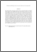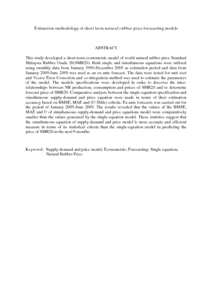# Estimation methodology of short term natural rubber price forecasting models

## Citation

Khin, Aye Aye and Mohamed, Zainal Abidin and Shamsudin, Mad Nasir and Chiew, Eddie Fook Chong and Mohamed, Fatimah (2011) Estimation methodology of short term natural rubber price forecasting models. Journal of Environmental Science and Engineering, 5 (4). pp. 460-474. ISSN 1934-8932; ESSN: 1934-8940

## Abstract

This study developed a short-term econometric model of world natural rubber price Standard Malaysia Rubber Grade 20(SMR20). Both single and simultaneous equations were utilized using monthly data from January 1990-December 2008 as estimation period and data from January 2009-June 2009 was used as an ex-ante forecast. The data were tested for unit root and Vector Error Correction and co-integration method was used to estimate the parameters of the model. The models specifications were developed in order to discover the inter-relationships between NR production, consumption and prices of SMR20 and to determine forecast price of SMR20. Comparative analysis between the single-equation specification and simultaneous supply-demand and price equation were made in terms of their estimation accuracy based on RMSE, MAE and (U-Thile) criteria. Ex-ante forecasts was carried out for the period of January 2009-June 2009. The results revealed that the values of the RMSE, MAE and U of simultaneous supply-demand and price equations model were comparatively smaller than the values generated by the single-equation model. These statistics suggest that the simultaneous equation of supply-demand and price model is more accurate and efficient measure in terms of its statistical criteria than the single-equation model in predicting the price of SMR20 in the next 6 months.Preview
PDF (Abstract)
Estimation methodology of short term natural rubber price forecasting models.pdfView Item